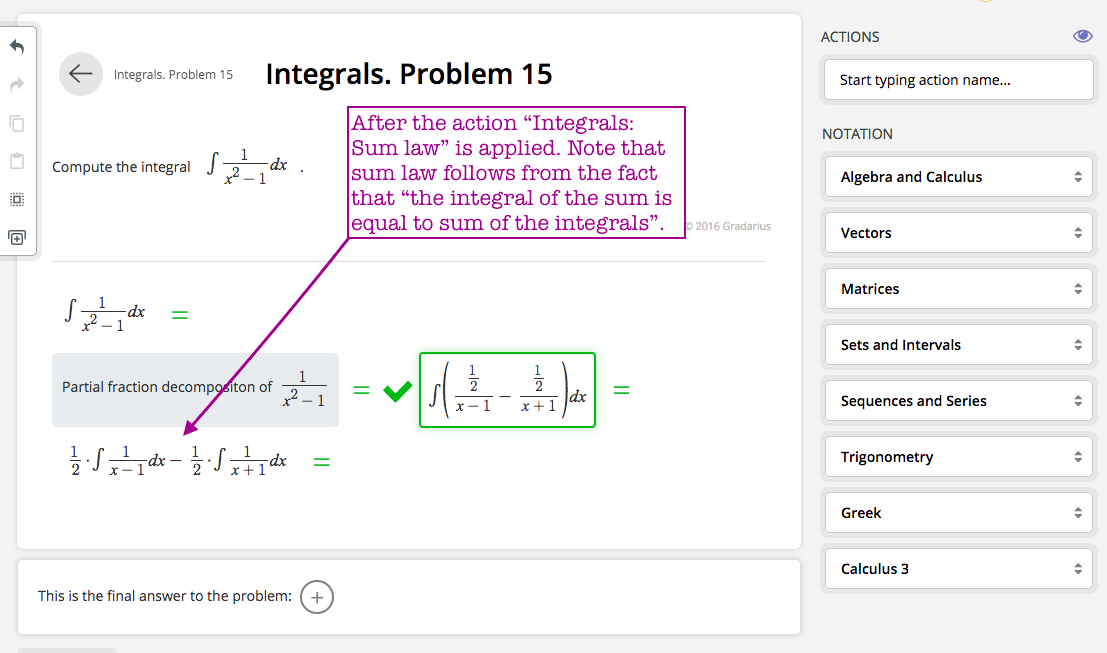This action is used when we need to divide an integral into two sub integrals. Note that sum law follows from the fact that the integral of sum of two functions is equal to sum of the integrals of the functions.

Please take a look at the screenshots below to see how this action is used in practice.